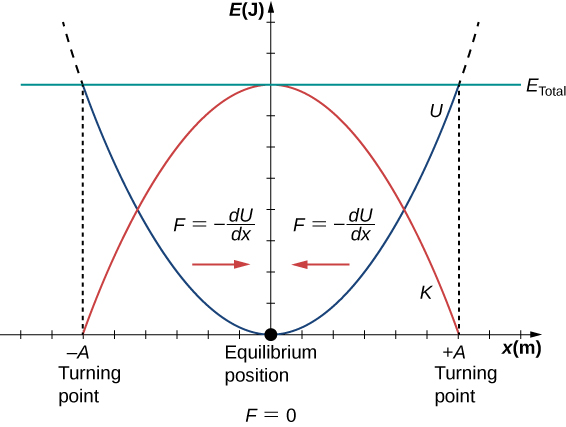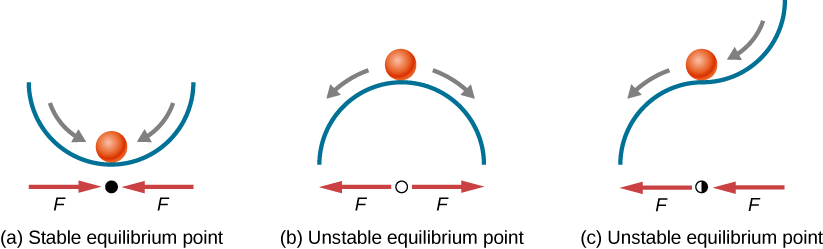# 15.2 Energy in simple harmonic motion  (Page 3/8)

 Page 3 / 8

## Oscillations about an equilibrium position

We have just considered the energy of SHM as a function of time. Another interesting view of the simple harmonic oscillator is to consider the energy as a function of position. [link] shows a graph of the energy versus position of a system undergoing SHM.A graph of the kinetic energy (red), potential energy (blue), and total energy (green) of a simple harmonic oscillator. The force is equal to F = − d U d x . The equilibrium position is shown as a black dot and is the point where the force is equal to zero. The force is positive when x < 0 , negative when x > 0 , and equal to zero when x = 0 .

The potential energy curve in [link] resembles a bowl. When a marble is placed in a bowl, it settles to the equilibrium position at the lowest point of the bowl $\left(x=0\right)$ . This happens because a restoring force    points toward the equilibrium point. This equilibrium point is sometimes referred to as a fixed point . When the marble is disturbed to a different position $\left(x=+A\right)$ , the marble oscillates around the equilibrium position. Looking back at the graph of potential energy, the force can be found by looking at the slope of the potential energy graph $\left(F=-\frac{dU}{dx}\right)$ . Since the force on either side of the fixed point points back toward the equilibrium point, the equilibrium point is called a stable equilibrium point    . The points $x=A$ and $x=\text{−}A$ are called the turning points . (See Potential Energy and Conservation of Energy .)

Stability is an important concept. If an equilibrium point is stable, a slight disturbance of an object that is initially at the stable equilibrium point will cause the object to oscillate around that point. The stable equilibrium point occurs because the force on either side is directed toward it. For an unstable equilibrium point, if the object is disturbed slightly, it does not return to the equilibrium point.

Consider the marble in the bowl example. If the bowl is right-side up, the marble, if disturbed slightly, will oscillate around the stable equilibrium point. If the bowl is turned upside down, the marble can be balanced on the top, at the equilibrium point where the net force is zero. However, if the marble is disturbed slightly, it will not return to the equilibrium point, but will instead roll off the bowl. The reason is that the force on either side of the equilibrium point is directed away from that point. This point is an unstable equilibrium point.

[link] shows three conditions. The first is a stable equilibrium point (a), the second is an unstable equilibrium point (b), and the last is also an unstable equilibrium point (c), because the force on only one side points toward the equilibrium point.Examples of equilibrium points. (a) Stable equilibrium point; (b) unstable equilibrium point; (c) unstable equilibrium point (sometimes referred to as a half-stable equilibrium point).

The process of determining whether an equilibrium point is stable or unstable can be formalized. Consider the potential energy curves shown in [link] . The force can be found by analyzing the slope of the graph. The force is $F=-\frac{dU}{dx}.$ In (a), the fixed point is at $x=0.00\phantom{\rule{0.2em}{0ex}}\text{m}\text{.}$ When $x<0.00\phantom{\rule{0.2em}{0ex}}\text{m,}$ the force is positive. When $x>0.00\phantom{\rule{0.2em}{0ex}}\text{m,}$ the force is negative. This is a stable point. In (b), the fixed point is at $x=0.00\phantom{\rule{0.2em}{0ex}}\text{m}\text{.}$ When $x<0.00\phantom{\rule{0.2em}{0ex}}\text{m,}$ the force is negative. When $x>0.00\phantom{\rule{0.2em}{0ex}}\text{m,}$ the force is also negative. This is an unstable point.

Damping is provided by tuning the turbulence levels in the moving water using baffles.How it happens? Give me a labelled diagram of it.
A 10kg ball travelling at 4meter per second collides elastically in a head-on collision with a 2kg ball.What are (a)the velocities and (b)the total momentum of the balls after collision?
a)v1 8/3s&v2 20/3s. b)in elastic collision total momentum is conserved.
Bala
The displacement of the air molecules in sound wave is modeled with the wave function s(x,t)=5.00nmcos(91.54m−1x−3.14×104s−1t)s(x,t)=5.00nmcos(91.54m−1x−3.14×104s−1t) . (a) What is the wave speed of the sound wave? (b) What is the maximum speed of the air molecules as they oscillate in simple harmon
practical 1st year physics
huh
Luminous
Whats the formular for newton law of motion
f=ma
F=m×a Where F=force M=mass of a body of an object a=acceleration due to gravity
Abubakar
what is speed
distance travelled per unit of time is speed.
distance travelled in a particular direction it is.
Andrew
Speed is define as the distance move per unit time. Mathematically is given as Speed = distance/time Speed = s/t
Abubakar
speed is a vector quantity. It is defined distance per unit time.It's unit in c.g.s cm/s and in S.I. m/s.It’s dimension is LT^-1
Mukulika
formula for velocity
v=ms^-1 velocity=distance time
Cleophas
(p-a/v)(v-b)=nrt what is the dimension of a
Amraketa
velocity= displacement time
Gold
Velocity = speed/time
Abubakar
what are evasive medical diagnosis?
If the block is displaced to a position y , the net force becomes Fnet=k(y−y0)−mg=0Fnet=k(y−y0)−mg=0 . But we found that at the equilibrium position, mg=kΔy=ky0−ky1mg=kΔy=ky0−ky1 . Substituting for the weight in the equation yields. Show me an equation of graph.
Shaina
simple harmonic motion defination
how to easily memorize motion equation
Maharam
how speed destrog is uranium
where can we find practice problems?
I'm not well
YAZID
Sayed
can u tell me the expression for radial acceleeation
No
YAZID
Is equal to the square of the velocity divided by the radius of circular path of the object
Mukhtaar
how to find maximum acceleration and velocity of simple harmonic motion?
chander
how to find maximum acceleration and velocity of simple harmonic motion and where it occurres?
chander
you can use either motion equations or kinetic equation and potential equation .
lasitha
how destraction 1kg uranium
Sayed
A Radial Acceleration is defined as the upward movement of an object.
Andrew
A body of 2.0kg mass makes an elastic collision with another at rest and continues to more in the original direction but with 1/4 of its ori is the mass of the struck body?
pls help me solve this problem
bright
why do sound travel faster in the night than in the day
I believe because speed is a function of air density, and colder air is more dense
Jerry
At night air is denser because of humidity.
Clifton
Night air is cooler. Sound requires medium to travel so the denser the medium the fastest the sound travels. Humid air is denser then warmer air as in day.
Clifton
The humidity statement is misleading , colder air is more dense period.
Jerry
because there is no any other sound to reverberate with it so it clearly travel to lot of distance and also humidity and also due to denser air at night
Azam
please could you guys help me with physics best websites
Baje
because it is quiet at night. this takes us to the topic wave, it depends on the wave at that moment, which Echo's....sound travelled.
Andrew
because it is quite at night. this takes us to the topic wave , it depends on the wave at that the physics
mehreen
element radioactivit diffusion atomic radius alpha beta gamma these elements have more than 92 atomic mass start from uranium
SayedBy RhodesByByByByBy Anonymous User